# HC Verma Solutions Class 11 Chapter 6 Friction

HC Verma Solutions class 11 Chapter 6 – Friction is provided here to help students learn and incorporate the best answers for questions that are asked in this unit. Every solution of HC Verma exercises is arranged in a systematic manner in order to give students an easy learning experience while solving the questions. As for the chapter, it consists of conceptual problems that are likely to be asked in prominent examinations. It is highly recommended that students should go through the solutions and practice solving the questions in order to develop better skills and prepare efficiently. Notably, HC Verma book is a highly recommended study tool to prepare efficiently during JEE Preparation. The chapter friction consists of questions that contain free body diagrams. Students need to get clarity on these types of questions. In essence, students can understand the concept clearly by referring the HC Verma Solutions for class 11 physics that consists of well-written solutions along with solved examples. Students can clear any of their doubts with respect to the topics discussed in the chapter and they can seemingly practise problems to master the subject.

Download All the Solutions As PDF: HC Verma Solutions Chapter 6 PDF

Students will also learn about important topics like;

1. Friction as the Component of Contact Force
2. Kinetic Friction
3. Static Friction
4. Laws of Friction
5. Understanding Friction at Atomic Level
6. A Laboratory Method to Measure
7. Friction Coefficient

## Class 11 Important Questions In Chapter 6

1. If you take a heavy box, do you feel that it is easy to push it from behind? Why is it hard to push from the top? Explain both the situations.
2. An athlete takes a walk for 1 km daily. Calculate his average friction force.
3. It is very slippery to stand or walk on solid ice? Explain.
4. There are two bikes which have unequal masses. However, they both have similar tyres. If they move with the same initial speed, what will happen to the minimum stopping distance? (a) It will be smaller for the lighter bike. (b) Smaller for the heavier bike. (c) The distance remains the same for both bikes. (d) The volume of the bike affects the stopping distance.
5. Suppose, two teams are playing tug of war on a frictionless surface. Will there be any winners?

## HC Verma Solutions Vol 1 Friction Chapter 6

Question 1: A body slipping on a rough horizontal plane moves with a deceleration of 4.0 m/s2. What is the coefficient of kinetic friction between the block and the plane?

Solution:

Given:

The deceleration of body due to friction (a) = 4.0 m/s2

Let m be the mass of the body and mg N be the weight of the body.

Frictional force (f)= ma

=> f = 4m N

Coefficient of kinetic friction = 4m/mg = 4/g = 0.4

Therefore, μ= 0.4

The co-efficient of kinetic friction between the block and the plane is 0.4.

Question 2: A block is projected along a rough horizontal road with speed of 10 m/s. if the coefficient of kinetic friction is 0.10, how far will it travel before coming to rest?

Solution:

Given,

Coefficient of kinetic friction = μ= 0.10

Initial velocity of the body = u = 10m/s

Let m be the mass of the body and mg N be the weight of the body

Normal force on the body N = mg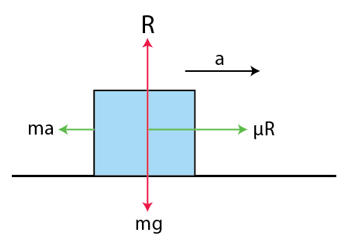Frictional force = f= μN = 0.10 mg

Deacceleration due to kinetic friction =a =force/mass

= (0.10 mg)/m = 0.10g= 0.98 m/s2

Final velocity due to deacceleration v= 0 m/s

Using equation, V2 = u2 + 2as, we have

0 = 102 + 2(0.98)s

=> s = 51 m

It will travel 51m before coming to rest.

Question 3: A block of mass m is kept on a horizontal table. If the static friction coefficient is μ, find the frictional force acting on the block.

Solution:

As the block is kept on horizonal surface and it is at rest, the frictional force will be zero. When the force is applied on the body to move it, the frictional force will act in opposite direction to oppose the motion. Hence, when body is at rest, frictional force will be zero.

Question 4: A block slides down an inclined surface of inclination 30o with the horizontal Starting from rest it covers 8 m in the first two seconds. Find the coefficient of kinetic friction between the two.

Solution:

Angle of inclination= 30o

Time taken (t)= 2 sec

Distance travelled (s) = 8m

Initial velocity of the body= 0 m/s

Let “a” be the acceleration of the body.

Using equation, s = ut + (1/2) at2

=> 8 = 0 + 1/2 a (22)

=> a = 4 m/s2

Now, the net force on the body = F = m.a = m (4)= 4m N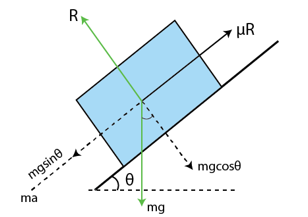Let us consider “f” frictional force experienced by body while moving

So, the net force on the body:

= mg sin30°- f

= (1/2) mg – f

On equating the above two equations, we get

(1/2) mg – f = 4m

=> f = 0.9 m

Normal force on the body= mg cos30° = √3/2 (9.8)m= 8.48m

Coefficient of kinetic energy= 0.9m/8.48m = 0.11

Therefore, the coefficient of kinetic friction between the surfaces is 0.11,

Question 5: Suppose the block of the previous problem is pushed down the incline with a force of 4 N. How far will the block move in the first two seconds after starting from rest? The mass of the block is 4 kg.

Solution:

Mass of the block = m = 4kg

When no external force was applied, net force is “mgsin30° – f”

Here, external force F = 0.9m

Total net force along the inclination = mg sin30° – f + 4 N

Substituting the values,

=> 4× 9.8 × 1/2 – (0.9 × 4)+ 4 N

= 20 N

Hence, the acceleration of the body = a =force/mass = 20/4 = 5 m/s2

The distance travelled in time 2 secs after starting from rest, s = ut + (1/2) at2

=> 0 + 1/2(5)(22) = 10 m

The block will move 10 m.

Question 6: A body of mass 2 kg is lying on a rough inclined plane of inclination 30o. Find the magnitude of the force parallel to the incline needed to make the block move (a) up the incline (b) down the incline. Coefficient of static friction = 0.2.

Solution:

mass of the body = m = 2kg

Angle of inclination = θ = 30°

Coefficient of static friction = μ = 0.2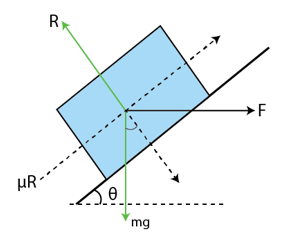(a)

To make the block move up the incline, the force should be equal and opposite to the net force acting down the incline i.e. μR + 2g sin 30o

= 0.2 x 9.8√3 + 2 x 9.8 x 1/2

= 13N

(b)

Net force acting down the incline

F = 2g sin 30o – μR

= 2 x 9.8 x 1/2 – 3.39

= 6.41 N

Due to force, F = 6.41 N, body will move in the incline with acceleration.

So, no external force is required. Therefore, force required is zero.

Question 7: Repeat part (a) of problem 6 if the push is applied horizontally and not parallel to the incline.

Solution:

mass of the body =m= 2kg

Angle of inclination =θ = 30o

Coefficient of static friction =μ = 0.2

and g = 10 m/s2from free body diagram,

R – mg cosθ – F sinθ = 0

or R = mg cosθ + F sinθ

and mg sin θ – μR – F cos θ = 0

Using value of R,

mg sin θ – μ(mg cosθ + F sinθ ) – F cos θ = 0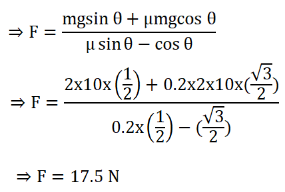Question 8: In a children-park an inclined plane is constructed with an angle of incline 45o in the middle part (figure below). Find the acceleration of a boy eliding on it if

the friction coefficient between the cloth of the boy and the incline is 0.6 and g = 10 m/s2.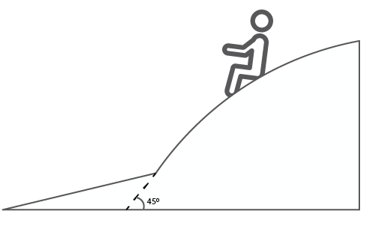Solution:

mass of the boy= m

Angle of inclination= θ = 45o

Coefficient of friction = μ = 0.6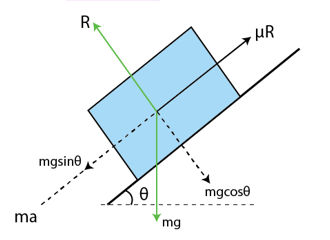R = mg cos(45o) = 0

=> R = mg/√2

Now, The force due to which boy is sliding down is

F = mg sin(45o) – μR

=> F = mg sin(45o) – μmg cos(45o)

=> F = m(10)(1/√2) – 0.6 m (10)(1/√2)

=> F = (2√2)m

Thus, Acceleration of the boy = a= force/mass = (2√2)m/m = (2√2) m/s2

Question 9: A body starts slipping down an incline and move, half meter in half second. How long will it take to move the next half meter?

Solution:

Let us consider “m” be the mass of the boy

Angle of inclination = θ

Acceleration of the body = a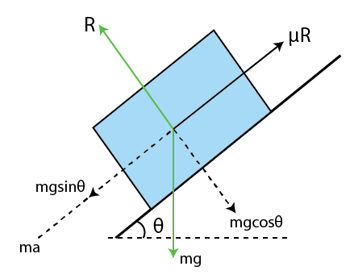Now,

R = mg cos θ …(1)

and ma = mg sin θ – μR …(2)

Using (1) in (2), we have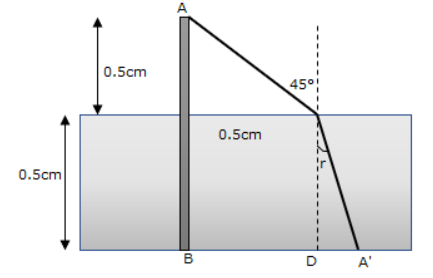Case 1:

In first half meter, the distance covered= s= 0.5 m

Time taken= t= 0.5sec

Initial velocity =u= 0

Using equation, s = ut+1/2 at2

=> (0.5) = 1/2 (a)(1/2) 2

=> a = 4 m/s2

case 2: For next half meter,

Velocity =u=2 m/s

Acceleration= a= 4 m/s2

Distance =s= 0.5m

Using equation of motion, s = ut + (1/2) at2

=> (0.5) = 2t + ( ½) (4)(t2)

=> t = 0.21 sec.

Question 10: The angle between the resultant contact force and the normal force exerted by a body on the other is called the angle of friction. Show that, if λ be the angle of friction and μ the coefficient of static friction, λ = tan-1 μ

Solution:

Let the frictional force to be f, and F be the applied force and normal reaction be R

Now, coefficient of friction force = μ = tan λ = f/R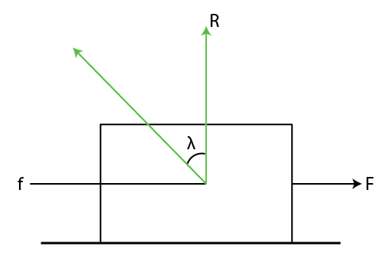When the force applied on the body increases, the force of friction also increases. It increases up to limiting friction. Before reaching to the limiting friction,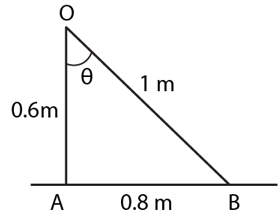Question 11: Consider the situation shown in figure (below). Calculate (a) the acceleration of the 1.0 kg blocks, (b) the tension in the string connecting the 1.0 kg block, and (c) the tension in the string attached to 0.50 kg.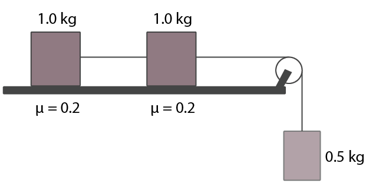Solution: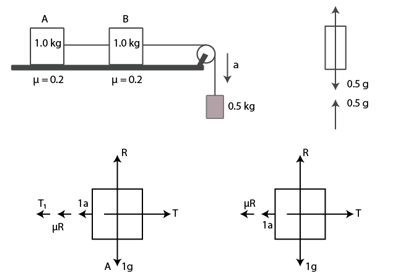From the free body diagram,

T + 0.5a – 0.5 g = 0 ..(1)

μR + a + T1 – T = 0 …(2)

μR + a = T1 …(3)

From equations (2) and (3

T = 2T1

(2)=> μR + a + T1 – 2 T1= 0

Or T1 = μR + a = 0.2 g + a …(4)

(1)=> 2T1 + 0.5a – 0.5g = 0

=> T1 = 0.25g – 0.25 a …(5)

From equations (4) and (5)

0.2g + a = 0.25g – 0.25a

=> a = 0.4 m/s2

Now,

(a) acceleration of 1 kg blocks each is 0.4 m/s2

(b) Tension T1 = 0.2g + a + 0.4 = 2.4N

(c) Tension = T = 0.5g – 0.5a = 4.8N

Question 12: If the tension in the string in figure (below) is 16 N and the acceleration of each block is 0.5 m/s2, find the friction coefficients at the two contacts with the blocks.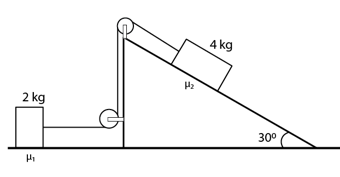Solution: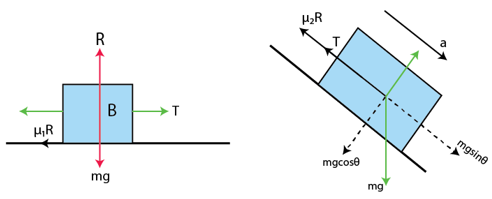From first figure:

μ1R + ma = T

μ1R + 2(0.5) = 16 {Here R = mg cosθ}

=>μ1 (2g) =15

=> μ1 = 0.75

From second figure:

μ2R + ma = F − mg sin θ

=>μ2mg cos θ + 4 (0.5) = 16 − 4g sin 30°

=> μ2 = 0.06

Question 13: The friction coefficient between the table and the block shown in figure (below) is 0.2. Find the tensions in the two strings.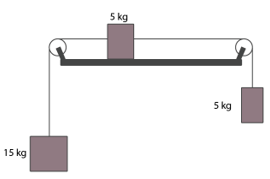Solution: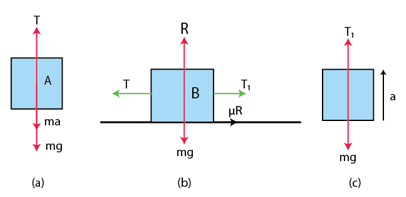Let us consider that the 15kg block is moving downward with the acceleration a.

Case 1: From figure (a)

T + ma − mg = 0

T + 15a − 15g = 0

⇒ T = 15g − 15a……. (1)

Case 2: From the figure (c)

T1 − mg − ma = 0

T1 − 5g − 5a = 0

⇒ T1 = 5g + 5a ………. (2)

Case 3: From figure (b)

T = (T1 + 5a + μR) = 0

⇒ T − (5g + 5a + 5a + μR) = 0 ……. (3)

(where R = μg)

From Equations (1) and (2),

15g − 15a = 5g + 10a + 0.2 (5g)

⇒ 25a = 90

⇒ a = 3.6 m/s2

From Equation (3),

T = 5 × 10 + 10 × 3.6 + 0.2 × 5 × 10

T= 96 N in the left string.

From Equation (2),

T1 = 5g + 5a

= 5 × 10 + 5 × 36= 50 + 18T1= 68 N in the right string.

Question 14: The friction coefficient between a road and the tyre of a vehicle is 4/3. Find the maximum incline the road may have so that once hard brakes are applied and the wheel starts skidding, the vehicle going down at a speed of 36 km/hr is stopped within 5 m.

Solution: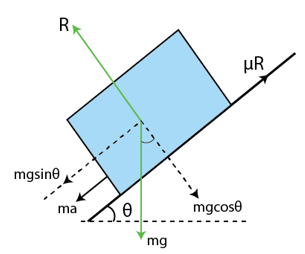Let θ the maximum angle of incline.

distance travel s = 5 m,

Initial velocity of the vehicle= u = 36 km/h = 10 m/s

Final velocity of the vehicle= v = 0

and μ=4/3, g = 10 m/s2

Now, V2 – u2 = 2as

r a = -10 m/s2

From the free body diagram

R = mg cosθ

Again, ma + mg sinθ = μR

=> ma + mg sinθ = μmg cosθ

=> a + g sinθ = μg cosθ

=> 10 +10 sinθ = 4/3×10 cosθ

=> 4 cosθ − 3 sin θ = 3

=> 4(1-sin2 θ)1/2 = 3 + 3 sinθ

=> 16 (1 − sin2 θ) = 9 + 9 sin2 θ + 18 sinθ

=> 25 sin2 θ + 18 sinθ − 7 = 0

or sinθ = 0.28

or θ = 16°

Maximum incline is θ = 16°

Question 15: The friction coefficient between an athelete’s shoes and the ground is 0.90. Suppose a superman wears these shoes and races for 50 m. There is no upper limit on his capacity of running at high speeds. (a) Find the minimum time that he will have to take in completing the 50 m starting from rest. (b) Suppose he takes exactly this minimum time to complete the 50 m, what minimum time will he take to stop?

Solution: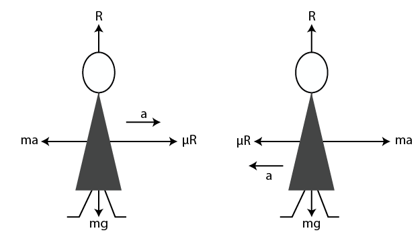Superman has to move with maximum possible acceleration, to reach the given distance in minimum time,

Let us consider “a” be the maximum acceleration.

So, ma − μR = 0

=> ma = μ mg

=> a = μg = 0.9 × 10 = 9 m/s2

(a)

In this case,

initial velocity =u = 0,

t = ?

acceleration =a = 9 m/s2,

distance s = 50 m

From the equation of motion,s = ut + (1/2) at2

50 = 0 + 1/2 x 9t2

or t = 10/3 sec

(b)

After covering 50 m, the velocity of the athlete

v = u + at

=0+9×103 m/s

=30 m/s

He has to stop in minimum time. Hence, the deceleration,

a =−9 m/s2 (max)

R = mg

ma = μR (maximum frictional force)

ma = μmg

⇒ a = μg

= 9 m/s2 (deceleration)

u1 = 30 m/s, v = 0

=> t = (v1-u1)/a

or t = 10/3 sec.

Question 16: A car is going at a speed of 21.6 km/hr when it encounters a 12.8 m long slope of angle 30o (figure below). The friction coefficient between the road and the tyre is 1/2√3. Show that no matter how hard the driver applies the brakes; the car will reach the bottom with a speed greater than 36 km/hr. Take g = 10 m/s2.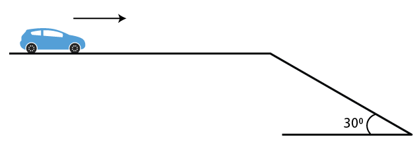Solution: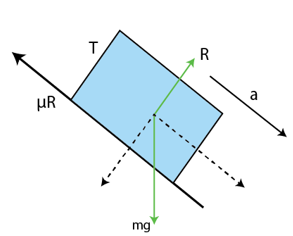Hardest brake means maximum force of friction is produced between car’s type and road.

maximum frictional force = μR

R − mg cos θ = 0

⇒ R = mg cos θ (i)

And μR + ma − mg sin θ = 0 (ii)

⇒ μ mg cos θ + ma − mg sin θ = 0

⇒ μg cos θ + a -10(12) =0

⇒ a = 5 – {1-(2√3)} ×10(√3/2)

or a = -2.5 m/s2

When brakes are applied, car will deaccelerate by 2.5 m/s2

Distance =s= 12.8 m

initial velocity= u = 6 m/s

∴ Velocity at the end of incline

v = √(u2+2as) = √(36+2(2.5)(12.8) = 36 km/h

Hence how hard the driver applies the breaks, car reaches the bottom with least velocity 36 km/h.

Question 17: A car starts from rest on a half kilometer long bridge. The coefficient of friction between the tyre and the road is 1.0. Show that one cannot drive through the bridge in less than 10 s.

Solution: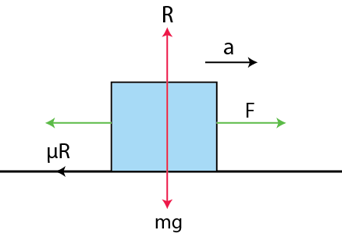From diagram,

ma = μR

ma = μmg

a = μg = 1 × 10 = 10 m/s2

To cross the bridge in minimum time, it must be at its maximum acceleration.

here, initial velocity u = 0, acceleration a = 10 m/s2 and Distance s = 500 m,

From equation s = ut + 1/2 at2

500 = 1/2 x 10 x 102

or t = 10 sec

car will take more than 10 sec to cross the bridge if the acceleration is less than 10 m/s2.

Question 18: Figure (Below) shows two blocks in contact sliding down an inclined surface of inclination 30o. The friction coefficient between the block of mass 2.0 kg and the incline is and that between the block of mass 4.0 kg and the incline is μ2. Calculate the acceleration of the 2.0 kg block if (a) μ1 = 0.20 and μ2 = 0.30, (b) μ1 = 0.30 and μ2 = 0.20. Take g = 10 m/s2.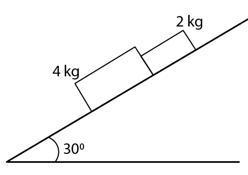Solution: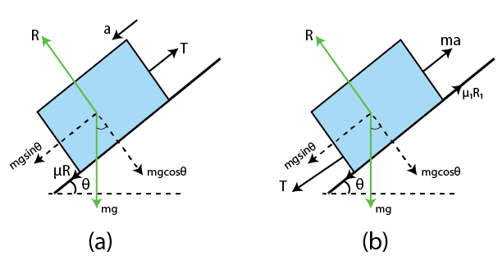Angle of inclination = θ = 30o

The free body diagram of the system is shown above

From the figure (a), mass = 4kg

R = 4g cos 30°

=> R = 20√3 N …(1)

and

μ2 R + ma = T + mg sin θ

μ2 R + 4a =T + 4g sin 30°

⇒ 0.3× (40) cos 30° + 4a = T + 40 sin 30° ….(2)

From the figure (a), mass = 2kg

R1 = 2g cos 30°

=10/√3 ….. (3)

T + 2a − μ1R1 − 2g sin 30° = 0 …..(4)

From Equation (2),

6√3 +4a-T-20=0

From Equation (4),

T+2a+2√3 -10=0

From equation (2) and (4)

6√3 + 6a – 30 + 2√3 = 0

=> 6a=30-8√3

=> a = 2.7 m/s2

(b) 4 kg block will move at a higher acceleration because the coefficient of friction is less than that of the 2 kg block. Therefore, the two blocks will move separately. By drawing the free body diagram of 2 kg mass, it can be shown that a = 2.4 m/s2.

Question 19: Two masses M1 and M2 are connected by a light rod and the system is slipping down a rough incline of angle θ with the horizontal. The friction coefficient at both the contacts is μ. Find the acceleration of the system and the force by the rod on one of the blocks.

Solution: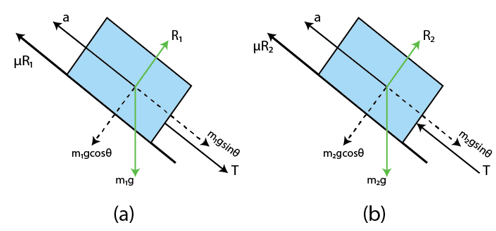R1 = m1g cos θ

T + m1sin θ = m1a+ μR1

T + m1g sin θ = m1a + μm1g cos θ ….. (i)

From figure (b)

R2 = m2g cos θ

T − m2g sin θ = m2a -μR2

T − m2g sin θ + m2a + μm2 gcos θ = 0 ….(ii)

From Equations (i) and (ii),

g sin θ(m1 + m2) − a(m1 + m2) − μg cos θ(m1 + m2) = 0

=> a = g(sin θ − μ cos θ)

Hence, the acceleration of the system = g(sin θ − μcos θ)

The force exerted by the rod on one of the blocks is tension, T.

T = m1g sin θ + m1a + μm1g cos θ

T = − m1g sin θ + m1(g sin θ − μg cos θ) + μm1g cos θ = 0

=> T=0

Question 20: A block of mass M is kept on a rough horizontal surface. The coefficient of static friction between the block and the surface is μ. The block is to be pulled by applying a force to it. What minimum force is needed to slide the block? In which direction should this force act?

Solution: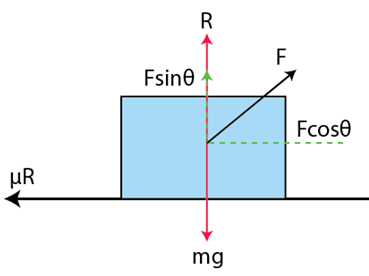From the free body diagram,

R + F sin θ = mg

⇒ R = −F sin θ + mg …(1)

μR = F cos θ …..(2)

From Equation (1),

μ(mg − Fsin θ)−F cos θ = 0

⇒ μmg = μF sin θ +F cos θ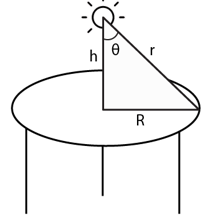F should be minimum, when μ sin θ + cos θ is maximum.

Again, μ sin θ + cos θ is maximum when its derivative is zero:

d/dθ (μsin θ+ cos θ) = 0

⇒ μ cos θ − sin θ = 0

θ = tan−1 μ

So,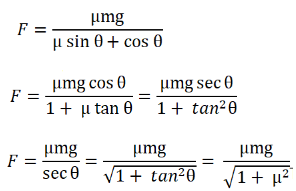Minimum force required is μmg/√(1+μ^2)

Question 21: The friction coefficient between the board and the floor shown in figure (below) is μ. Find the maximum force that the man can exert on the rope so that the board does not slip on the floor.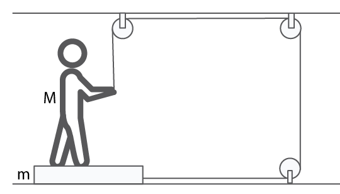Solution: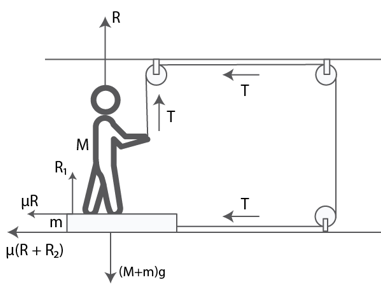R + T = Mg

⇒ R = Mg − T ….(1)

Also,R1 − R − mg = 0

⇒ R1 = R + mg …..(2)

And T − μR1 = 0

From Equation (2),

T − μ(R + mg) = 0

⇒ T − μR − μ mg = 0

⇒ T − μ(Mg − T) − μmg = 0

T − μMg + μt − μmg = 0

⇒ T (1 + μ) = μMg + μmg

=> T = (μ(M+m)g)/(1+μ), which is the maximum force exerted by the man.

Question 22: A 2 kg block is placed over a 4 kg block and both are placed on a smooth horizontal surface. The coefficient of friction between the blocks is 0.20. Find the acceleration of the two blocks if a horizontal force of 12 N is applied to (a) the upper block, (b) the lower block. Take g = 10 m/s2.

Solution: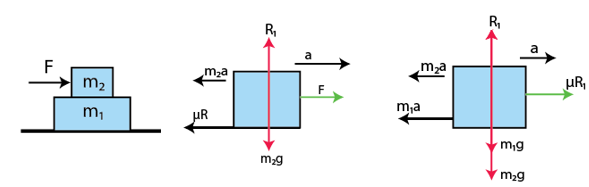for the mass of 2 kg m2

R1 − 2g = 0

⇒ R1 = 2 × 10 = 20

2a + 0.2 R1 − 12 = 0

⇒ 2a + 0.2 (20) = 12

⇒ 2a = 12 − 4

⇒ a = 4 m/s2

for 4kg block mass m1

4a − μR1 = 0

⇒ 4a = μR1 = 0.2 (20) = 4

⇒ a = 1 m/s2

The 2 kg block has acceleration 4 m/s2 and the 4 kg block has acceleration 1 m/s2.

we can also write,

R1 = 2g = 20

Ma = μR1 = 0

a = 0

and

Ma + μmg − F = 0

4a + 0.2 × 2 × 10 − 12 = 0

⇒ 4a + 4 = 12

⇒ 4a = 8

⇒ a = 2 m/s2

Question 23: Find the accelerations a1, a2, a3 of the three blocks shown in figure (below) if a horizontal force of 10 N is applied on (a) 2 kg block, (b) 3 kg block, (c) 7 kg block. Take g = 10 m/s2.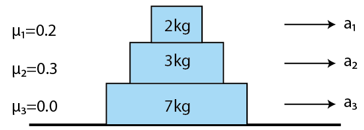Solution: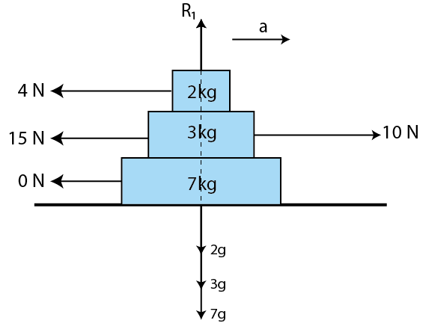The coefficient of frictions are given as, μ1 = 0.2, μ2 = 0.3 and μ3 = 0.4

(a) When the 10 N force is applied to the 2 kg block, it experiences maximum frictional force.

Here,μ1R1 = μ1 × m1g

μ1R1 = μ1 × 2g

= (0.2) × 20

= 4 N (From the 3 kg block)

The net force experienced by the 2 kg block = 10 – 4 = 6 N

Hence, a1 = 6/2 = 3 m/s2

In this case, the frictional force from the 2 kg block becomes the driving force (4N) and the maximum frictional force between the 3 kg and 7 kg blocks.

so, μ2R2 = μ2m2g = (0.3) × 5 kg = 15 N

3 kg block cannot move relative to the 7 kg block, because there is no friction from the floor.

So, a2 = a3 = 4/10 = 0.4 m/s2

(b) When the 10 N force is applied to the 3 kg block, it experiences maximum frictional force of 19 N, from 2 kg and 7 kg block. As the floor is frictionless, all the three bodies will move together.

a = 10/12 = 5/6 m/s2

(c) when 10 N force is applied to the 7 kg block, all three blocks will move together with the same acceleration.

Hence, a1 = a2 = a3 = 5/6 m/s2

Question 24: The friction coefficient between the two blocks shown in figure (6-E9) is μ but the floor is smooth. (a) What maximum horizontal force F can be applied without disturbing the equilibrium of the system? (b) Suppose the horizontal force applied is double of that found in part (a). Find the accelerations of the two masses.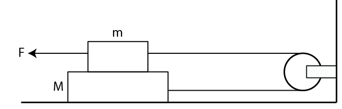Solution: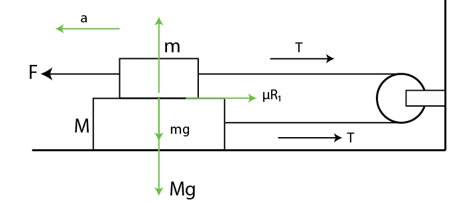(a) R1 = mg …(i)

F = μR1+T …(ii)

T − μR1 = 0 ..(iii)

From equations (i) and (ii)

F − μmg = T …(ii)

From equations (i) and (iii)

T = μmg

Putting T = μmg in equation (ii)

F = μmg + μmg = 2μmg

(b)

From upper block of free body, we have

2F − T − μmg = ma

From lower block of free body, we have

T = Ma + μmg

Equating both the equations,

2F − Ma − μmg − μmg = ma

Putting F = 2μmg, we get

2(2μmg) − 2μmg = a(M + m)⇒ 4μmg − 2μmg = a(M + m)

=> a = 2μmg/(M+m) in opposite directions.

Question 25: Suppose the entire system of the previous question is kept inside an elevator which is coming down with an acceleration a < g. Repeat parts (a) and (b).

Solution:

Referring Question 24 image.

(a)

R1 + ma − mg = 0

⇒ R1 = m (g − a)

= mg − ma

Now,F − T − μR1 = 0 and

T − μR1 = 0

⇒ F − [μ (mg − ma)] − μ(mg − ma) = 0

⇒ F − μ mg − μma − μmg + μma = 0

⇒ F = 2 μmg − 2 μma

= 2 μm (g − a)

(b) Let a1 be acceleration of the blocks, then

R1 = mg − ma ….(i)

2F −T − μR1 = ma1 …(ii)

Now, T = μR1 + Ma1

= μmg − μma + Ma1

Substituting the value of F and T in equation (ii),

2[2μm(g − a)] − (μmg − μma + Ma1) − μmg + μma = ma1

⇒ 4μmg − 4μma − 2μmg + 2μma= ma1 + Ma1

=>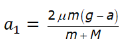Both the blocks move with same acceleration a1 but in opposite directions.

Question 26: Consider the situation shown in figure (below). Suppose a small electric field E exists in the space in the vertically upward direction and the upper block carries a positive charge Q On its top surface. The friction coefficient between the two blocks is μ but the floor is smooth. What maximum horizontal force F can be applied without disturbing the equilibrium?

[Hint: The force on a charge Q by the electric field E is F = QE in the direction of E.]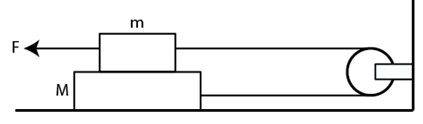Solution: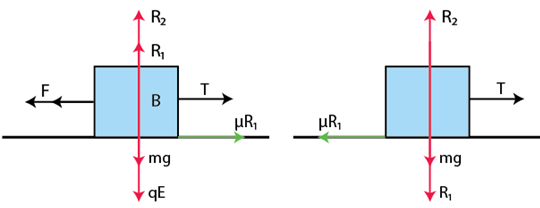R1 is the normal reaction force

E is the small electric field

Q is the charge

From the figure (b)

R1 + qE = mg

=> R1 = mg – qE …(1)

From figure (a)

F – T – μR1 = 0

=> F – T – μmg + μqE = 0 ….(2)

[Using (1)]

Again from (1) and (2)

T – μR1 = 0

=> T – μR1 = μ(mg – qE) = μmg – μqE

From (2),

F – μmg + μqE – μmg + μqE = 0

=> F = 2μ(mg – qE)

Which is the maximum horizontal force applied.

Question 27: A block of mass m slips on a rough horizontal table under the action of a horizontal force applied to it. The coefficient of friction between the block and the table is μ. The table does not move on the floor. Find the total frictional force applied by the floor on the legs of the table. Do you need the friction coefficient between the table and the floor or the mass of the table?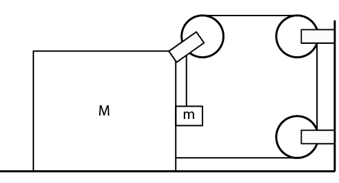Solution:

When the body is slipping from the surface, the maximum frictional force is acting on it.

R = mg

F − μR = 0

=> F = μR = μmg

As, table is at rest, the frictional force at table’s legs will also be μR. Let the frictional force be f,

f − μR = 0

⇒ f = μR = μmg

Therefore, the total frictional force on the table by the floor is μmg.

Question 28: Find the acceleration of the block of mass M in the situation of figure (6-E10). The coefficient of friction between the two blocks is μ1 and that between the bigger block and the ground is μ2.

Solution:

Let the acceleration of block of mass M is ‘a’ towards right. So, the block ‘m’ must go down with an acceleration ‘2a’. As the block ‘m’ is in contact with the block ‘M’, it will also have acceleration ‘a’ towards right.

R1 – ma = 0

⇒ R1 = ma ….(i)

Also,

2ma + T – Mg + μ1R1 = 0

⇒ T = Mg – (2 + μ1) ma …..(ii)

Also, T + μ1R1 + Mg – R2 = 0

using (i), R2 = T + μ1 ma + mg

again using value of T from (2), we get

R2 = Mg + Ma – 2ma …(iii)

Again, from figure we have

T + T – R – Ma – μ2R2 = 0

⇒ 2T – Ma – ma – μ2(Mg + mg – 2ma) = 0

Substituting the values of R1 and R2 from (i) and (iii), we get:

2T = (M + m)a + μ2 (Mg + mg – 2ma) ….(iv)

From equations (ii) and (iv), we have:

=> 2mg – μ2 (M + m)g = a[M + m – 2μ2m + 4m + 2μ1m]

Thus, acceleration of the block of mass M is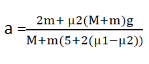Question 29: A block of mass 2 kg is pushed against a rough vertical wall with a force of 40 N, coefficient of static friction being 05. Another horizontal force of 15 N, is applied on the block in a direction parallel to the wall. Will the block move? If yes, in which direction? If no, find the frictional force exerted by the wall on the block.

Solution:

Net force on the block = (20)2 + (15)2 – 0.5 × 40 = 5 N

Therefore, tan θ = 20/15 = 4/3

or, θ = tan-1 (4/3) = 53°

The block will move at 53° angle with the 15 N force.

Question 30: A person (40 kg) is managing to be at rest between two vertical walls by pressing one wall A by his hands and feet and the other wall B by his back (figure 6-E11). Assume that the friction coefficient between his body and the walls is 0.8 and that limiting friction acts at all the contacts. (a) Show that the person pushes the two walls with equal force. (b) Find the normal force exerted by either wall on the person.

Take g = 10 m/s2.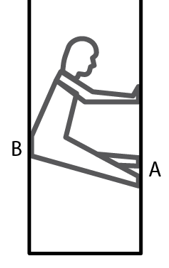Solution:

Mass of man = 50 kg and g = 10 m/s2

Frictional force developed between hands, legs & back with the wall will be equal to the weight of the man. Man remains in equilibrium.

If man applies unequal forces on the wall, the reaction force will be different and he can’t rest between the walls. Frictional force 2μR balance his body weight.

μR + μR = mg

=> 2μR = 40 × 10

=> R = 40 × 10/2 × 0.8 = 250 N, Which is normal force.

Question 31: Figure (Below) shows a small block of mass m kept at the left end of a larger block of mass M and length l. The system can slide on a horizontal road. The system is started towards right with an initial velocity u. The friction coefficient between the road and the bigger block is i and that between the block is μ/2. Find the time elapsed before the smaller blocks separates from the bigger block.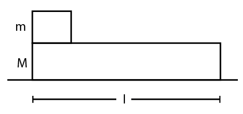Solution:

Let masses m and M are having acceleration a1 and a2 respectively.

if a1 > a2 so, mass m can move on mass M.

Also, consider after time ‘t’, the mass m separates from mass M

During this time, mass m covers the distance s,

s = vt + 1/2 a1t2

and s_m = ut + 1/2 a2 t2

For mass m to separate from mass M,

vt + 1/2 a1t2 = vt + 1/2 a2t2 …..(1)

ma1 + (1/2)μR = 0

ma1 = -(1/2)μmg = (1/2)μm × 10

a1 = -5μ

Again, from figure, Ma2+μ(M+m) g-(μ/2) mg = 0

2Ma2 = μmg − 2μmg − 2μmg

=> a2 = (-μmg – 2μMg)/2M

Substituting the values of a1 and a2 in equation (1), we get:

t = √[(4ml)/(M+m)μg]